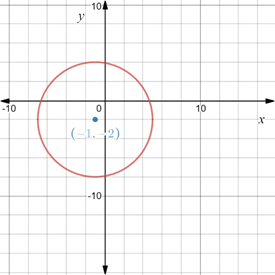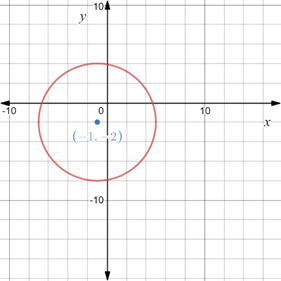# Thecenter and radius of the circle ( x + 1 ) 2 + ( y + 2 ) 2 = 36 and sketch the graph of the circle.### Precalculus: Mathematics for Calcu...

6th Edition
Stewart + 5 others
Publisher: Cengage Learning
ISBN: 9780840068071### Precalculus: Mathematics for Calcu...

6th Edition
Stewart + 5 others
Publisher: Cengage Learning
ISBN: 9780840068071

#### Solutions

Chapter 1.8, Problem 92E
To determine

## To calculate: Thecenter and radius of the circle (x+1)2+(y+2)2=36 and sketch the graph of the circle.

Expert Solution

The center of the circle is (1,2) and radius is 6 units. The graph of the circle is provided below,### Explanation of Solution

Given information:

The equation (x+1)2+(y+2)2=36 .

Formula used:

The standard form of the equation of the circle is (xh)2+(yk)2=r2 , where (h,k) denote the center of the circle and r denote the radius.

Calculation:

Consider the equation (x+1)2+(y+2)2=36 .

Rewrite the equation as,

(x(1))2+(y(2))2=62

Recall that the standard form of the equation of the circle is (xh)2+(yk)2=r2 , where (h,k) denote the center of the circle and r denote the radius.

Compare, (xh)2+(yk)2=r2 and (x(1))2+(y(2))2=62 .

Here, h=1,k=2 and r=6 .

Therefore, center of circle is (1,2) and radius is 6 .

Now, on the Cartesian plane construct a circle with center at (1,2) and radius 6 units.

The graph obtained is provided below,Thus, the center of the circle is (1,2) and radius is 6 units.

### Have a homework question?

Subscribe to bartleby learn! Ask subject matter experts 30 homework questions each month. Plus, you’ll have access to millions of step-by-step textbook answers!